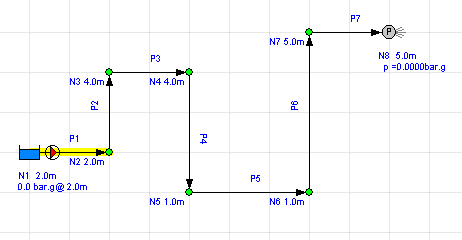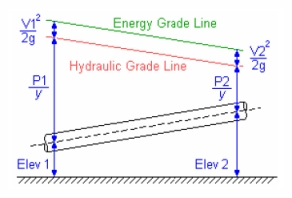# Pipe Elevation Changes and Effect on Pressure Loss

As fluid flows through a piping system, where pipes rise and fall, changing elevation, the pressure at a particular point in a pipe is also affected by the changes in elevation of the fluid that have occured.

For example, consider a single vertical pipe where the fluid is flowing upwards, gaining elevation height as it goes. The weight of fluid acting 'on top' of the fluid at a point in the pipe reduces as we consider points higher up the pipe, since there is less fluid above it. Therefore there is a loss of pressure in the pipe as the fluid rises.

Conversely, at the bottom of the vertical pipe there is the full weight of fluid in the pipe 'pushing down' on that point and due to this, the pressure at that point increases (in comparison to the pressure on the fluid at the top of the pipe). Therefore there is a gain in pressure in the pipe as the fluid falls.

## Flow through Multiple Changes of Elevation

As described above, the pressure on a fluid at a point in a piping run changes with the elevation of the fluid. As the fluid rises there is a pressure loss and as it falls there is an equivalent pressure gain (for the same change in elevation). Therefore we only need to consider the net change in fluid elevation between the start and end point of flow, to calculate the pressure loss/gain due to the elevation change.If fluid enters a run of pipe which has starting elevation of say 2m (relative to some zero point) and it then flows through the pipe system, rising and falling many times, before it finally exits at an elevation of say 5m, then the net change in elevation is 3m (5m - 2m), and the result will be a pressure loss due to change in elevation of of 3m fluid head (which could be converted to units of bar or psi, if required).

Of course there would also be pressure losses due to pipe friction, and in the diagram above, the pump would need to produce enough additional fluid head (pressure) to overcome both the pressure loss due to the change in elevation and the pressure loss due to pipe friction.

The Energy Grade Line, also called the Energy Line (EL), is a plot of the Bernoulli equation or the sum of three terms in the work-energy equation. The EL is equal to the sum of the fluid's velocity head, the pressure, and the elevation head.

EL = (V²/2g) + (p/γ) + h

where
V = velocity
g = acceleration due to gravity
p =static pressure (relative to the moving fluid)
γ = specific weight
h = elevation height

A pitot tube can be inserted into a pipe such that the fluid initially flows into the tip of the tube, until the height of fluid in the tube balances the energy coming in, at which point the flow in to the tube stops and the fluid velocity at the very tip of the pitot tube becomes zero. The pressure and the velocity head of the fluid are in effect converted to the equivalent head in height of fluid (i.e. the fluid will rise to the elevation of the EL for that specific point in the flow).

The Hydraulic Gradeline (HGL), is the sum of the pressure and elevation heads. This sum is known as the piezometric head and can be measured by inserting a piezometer tube into the side of a pipe so that it is flush with the edge of the pipe.

HGL = (p/γ) + h

where
p =static pressure (relative to the moving fluid)
γ = specific weight
h = elevation height

The HGL and EL of flow in a pipe can be illustrated on a diagram, with the pipe drawn at an angle and the associated elevation, pressure, and velocity heads marked up on the vertical axis at each end of the pipe. The resulting HGL and EL lines can then be drawn between specific points on each vertical axis to give a good visualisation of how these change for fluid flow at different points along the pipe.Secure Online PaymentsPipe Flow Software: Piping design, Pressure drop calculator, Flow rate calculator, Pump head calculations, Pump selection software.  Copyright © Pipe Flow Software 1997-2020